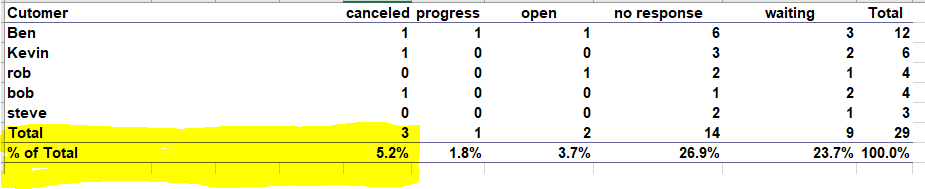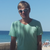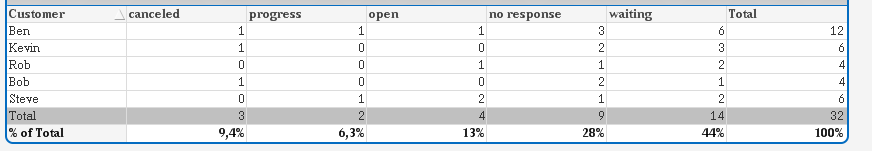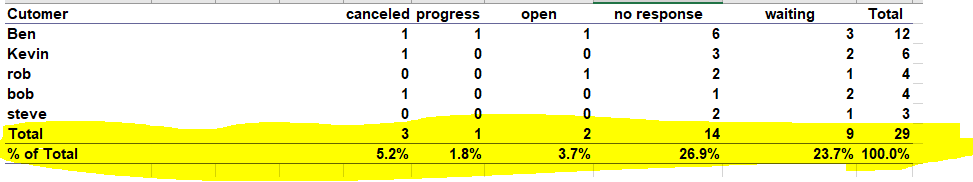# QlikView App Dev

Discussion Board for collaboration related to QlikView App Development.

Announcements
QlikWorld 2023, a live, in-person thrill ride. Save \$300 before February 6: REGISTER NOW!
cancel
Showing results for
Did you mean:Creator III

## Totals and % totals in Qlikview straight table

Hi all,

Is there any way around to show totals and % of totals in straight table as shown in below imageCan any one help.

Thanks,

Ganesh

1 Solution

Accepted SolutionsMaster II

```LOAD * INLINE [
Dim
1
2
];

Customer, Progress, Open, No Response, Waiting, Canceled
Ben, 1, 1, 6, 3, 1
Kevin, 0, 0, 3, 2, 1
Rob, 0, 1, 2, 1, 0
Bob, 0, 0, 1, 2, 1
Steve, 1, 2, 2, 1, 0
Ok];
```

Dimension:

```=Pick(Dim,Customer,'Total')
```

Expression 1 for canceled:

```if(Dimensionality()=0,
num(sum(Canceled)/(sum(Canceled)+sum(Progress)+sum(Open)+sum([No Response])+sum(Waiting)),'##%'),
sum(Canceled))
```

Expression 2 for progress:

```if(Dimensionality()=0,
num(sum(Progress)/(sum(Canceled)+sum(Progress)+sum(Open)+sum([No Response])+sum(Waiting)),'##%'),
sum(Progress))
```

Expression 3 for open:

```if(Dimensionality()=0,
num(sum(Open)/(sum(Canceled)+sum(Progress)+sum(Open)+sum([No Response])+sum(Waiting)),'##%'),
sum(Open))
```

Expression 4 for no response:

```if(Dimensionality()=0,
num(sum(Waiting)/(sum(Canceled)+sum(Progress)+sum(Open)+sum([No Response])+sum(Waiting)),'##%'),
sum(Waiting))
```

Expression 5 for waiting:

```if(Dimensionality()=0,
num(sum([No Response])/(sum(Canceled)+sum(Progress)+sum(Open)+sum([No Response])+sum(Waiting)),'##%'),
sum([No Response]))
```

Expression 6 for TOTAL:

```if(Dimensionality()=0,
num(Column(1)+Column(2)+Column(3)+Column(4)+Column(5),'###%'),
Column(1)+Column(2)+Column(3)+Column(4)+Column(5))
```

And this is my Output:hope this helps!

12 RepliesMaster II

see attached file,

hope this helpsCreator III
Author

Hi Frank,

I can not open the qvw files, could you please share any useful community links.

Thanks,Master II

Skript:

```LOAD * INLINE [
Dim
1
2
];

Customer, Progress, Open, No Response, Waiting, Canceled
Ben, 1, 1, 6, 3, 1
Kevin, 0, 0, 3, 2, 1
Rob, 0, 1, 2, 1, 0
Bob, 0, 0, 1, 2, 1
Steve, 1, 2, 2, 1, 0
];
```

As dimension in your straight table use:

=Pick(Dim,Customer,'% of Total')

Expressions:

if(Pick(Dim,Customer,'% of Total')='% of Total',

num(sum(Canceled)/(sum(Canceled)+sum(Progress)+sum(Open)+sum([No Response])+sum(Waiting)),'##%'),

sum(Canceled))

if(Pick(Dim,Customer,'% of Total')='% of Total',

num(sum(Progress)/(sum(Canceled)+sum(Progress)+sum(Open)+sum([No Response])+sum(Waiting)),'##%'),

sum(Progress))

if(Pick(Dim,Customer,'% of Total')='% of Total',

num(sum(Open)/(sum(Canceled)+sum(Progress)+sum(Open)+sum([No Response])+sum(Waiting)),'##%'),

sum(Open))

if(Pick(Dim,Customer,'% of Total')='% of Total',

num(sum(Waiting)/(sum(Canceled)+sum(Progress)+sum(Open)+sum([No Response])+sum(Waiting)),'##%'),

sum(Waiting))

if(Pick(Dim,Customer,'% of Total')='% of Total',

num(sum([No Response])/(sum(Canceled)+sum(Progress)+sum(Open)+sum([No Response])+sum(Waiting)),'##%'),

sum([No Response]))

if(Pick(Dim,Customer,'% of Total')='% of Total',

num(Column(1)+Column(2)+Column(3)+Column(4)+Column(5),'###%'),

Column(1)+Column(2)+Column(3)+Column(4)+Column(5))

hope this helpsMaster II

and for sorting use this expression:

only({1}Dim)  --> AscendingMaster II

Do you have any further questions?Creator III
Author

Thanks for the valuable reply, but total count row is missing form your example how to get that?

and also % symbol is missing form my % of total rows. FYI I am using 'dimensionality()=0' instead Pick(Dim,Customer,'% of Total')='% of Total',

Thanks,

GaneshCreator III
Author

I have above mentioned question on

'total count row is missing form your example how to get that?'

sure if i am clear, will close the thread.

Thanks,

GaneshMaster II

```LOAD * INLINE [
Dim
1
2
];

Customer, Progress, Open, No Response, Waiting, Canceled
Ben, 1, 1, 6, 3, 1
Kevin, 0, 0, 3, 2, 1
Rob, 0, 1, 2, 1, 0
Bob, 0, 0, 1, 2, 1
Steve, 1, 2, 2, 1, 0
Ok];
```

Dimension:

```=Pick(Dim,Customer,'Total')
```

Expression 1 for canceled:

```if(Dimensionality()=0,
num(sum(Canceled)/(sum(Canceled)+sum(Progress)+sum(Open)+sum([No Response])+sum(Waiting)),'##%'),
sum(Canceled))
```

Expression 2 for progress:

```if(Dimensionality()=0,
num(sum(Progress)/(sum(Canceled)+sum(Progress)+sum(Open)+sum([No Response])+sum(Waiting)),'##%'),
sum(Progress))
```

Expression 3 for open:

```if(Dimensionality()=0,
num(sum(Open)/(sum(Canceled)+sum(Progress)+sum(Open)+sum([No Response])+sum(Waiting)),'##%'),
sum(Open))
```

Expression 4 for no response:

```if(Dimensionality()=0,
num(sum(Waiting)/(sum(Canceled)+sum(Progress)+sum(Open)+sum([No Response])+sum(Waiting)),'##%'),
sum(Waiting))
```

Expression 5 for waiting:

```if(Dimensionality()=0,
num(sum([No Response])/(sum(Canceled)+sum(Progress)+sum(Open)+sum([No Response])+sum(Waiting)),'##%'),
sum([No Response]))
```

Expression 6 for TOTAL:

```if(Dimensionality()=0,
num(Column(1)+Column(2)+Column(3)+Column(4)+Column(5),'###%'),
Column(1)+Column(2)+Column(3)+Column(4)+Column(5))
```

And this is my Output:hope this helps!Creator III
Author

If i use new expressions, i am getting out put like this.But i  need output like this, I need both the rows Total and '% of Total'Thanks,

GaneshCommunity Browser